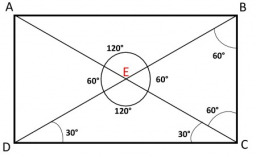# Rectangle 62854

The aspect ratio of the rectangle and its diagonal is 9:12:15. Calculate the area of the rectangle if the length of the diagonal is 105 cm.

a =  63 cm
b =  84 cm

### Step-by-step explanation:Did you find an error or inaccuracy? Feel free to write us. Thank you!

Tips for related online calculators
Check out our ratio calculator.
Do you want to convert length units?